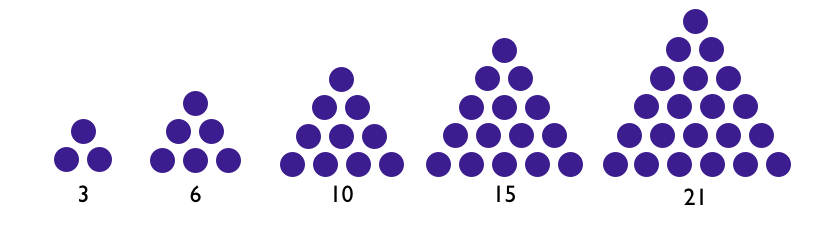# Triangular NumbersThe following statement is to be proven or disproven:

Let $T(n)=\frac{n(n+1)}{2}$ denote the triangular numbers.

If $AT(n)+B$ is also a triangular number for all integer values $(A,B)$ then $A$ is a perfect square, and $B$ is a triangular number.

Can $A$ be a perfect square of an even number?

Solution

If $AT(n)+B$ is a triangular number, we may generalize the formula as $AT(n)+B = \frac{(an+p)(an+p+1)}{2}$ where $a,n,p$ are natural numbers.

Substitute the above expression for $T(n)$ to the LHS and expand the RHS. Multiply both sides by 2 to get

$A{n}^{2}+An+2B = {a}^{2}{n}^{2}+a(2p+1)n+p(p+1).$

By equating like terms on both sides, we discover that A is a perfect square and B is a triangular number:

$A = {a}^{2} = a(2p+1)$

$B = \frac{p(p+1)}{2}.$

Since $A = {a}^{2} = a(2p+1)$, we find that $a = 2p+1$, an odd number.

Therefore, $A$ is a perfect square of an odd number, and $B$ is a triangular number.

* As an exercise, prove that $A = 1+ 8B$.*

Check out my other notes at Proof, Disproof, and DerivationNote by Steven Zheng
7 years ago

This discussion board is a place to discuss our Daily Challenges and the math and science related to those challenges. Explanations are more than just a solution — they should explain the steps and thinking strategies that you used to obtain the solution. Comments should further the discussion of math and science.

When posting on Brilliant:

• Use the emojis to react to an explanation, whether you're congratulating a job well done , or just really confused .
• Ask specific questions about the challenge or the steps in somebody's explanation. Well-posed questions can add a lot to the discussion, but posting "I don't understand!" doesn't help anyone.
• Try to contribute something new to the discussion, whether it is an extension, generalization or other idea related to the challenge.

MarkdownAppears as
*italics* or _italics_ italics
**bold** or __bold__ bold
- bulleted- list
• bulleted
• list
1. numbered2. list
1. numbered
2. list
Note: you must add a full line of space before and after lists for them to show up correctly
paragraph 1paragraph 2

paragraph 1

paragraph 2

[example link](https://brilliant.org)example link
> This is a quote
This is a quote
    # I indented these lines
# 4 spaces, and now they show
# up as a code block.

print "hello world"
# I indented these lines
# 4 spaces, and now they show
# up as a code block.

print "hello world"
MathAppears as
Remember to wrap math in $$ ... $$ or $ ... $ to ensure proper formatting.
2 \times 3 $2 \times 3$
2^{34} $2^{34}$
a_{i-1} $a_{i-1}$
\frac{2}{3} $\frac{2}{3}$
\sqrt{2} $\sqrt{2}$
\sum_{i=1}^3 $\sum_{i=1}^3$
\sin \theta $\sin \theta$
\boxed{123} $\boxed{123}$

Sort by:- 6 years, 6 months ago

Solution

If $AT(n)+B$ is a triangular number, we may generalize the formula as $AT(n)+B = \frac{(an+p)(an+p+1)}{2}$ where $a,n,p$ are natural numbers.

Substitute the above expression for $T(n)$ to the LHS and expand the RHS. Multiply both sides by 2 to get

$A{n}^{2}+An+2B = {a}^{2}{n}^{2}+a(2p+1)n+p(p+1).$

By equating like terms on both sides, we discover that A is a perfect square and B is a triangular number:

$A = {a}^{2} = a(2p+1)$

$B = \frac{p(p+1)}{2}.$

Since $A = {a}^{2} = a(2p+1)$, we find that $a = 2p+1$, an odd number.

Therefore, $A$ is a perfect square of an odd number, and $B$ is a triangular number.

* As an extra exercise, prove that $A = 1+ 8B$.*

- 6 years, 11 months ago